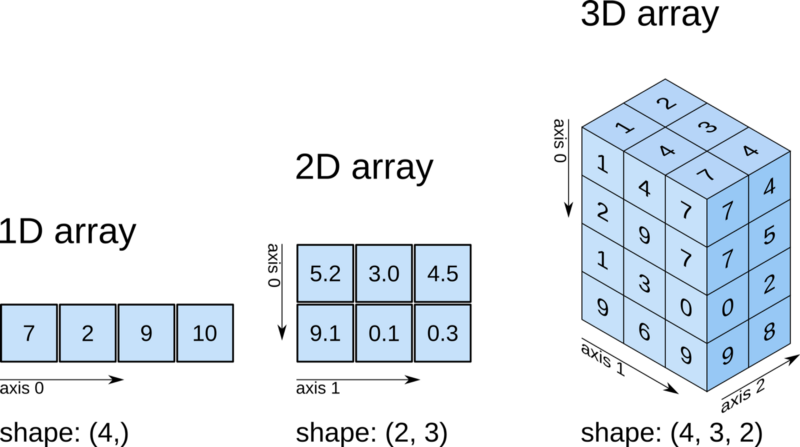# 一份精简的Numpy使用指引（附python演练）

Numpy 的主要用途是以数组的形式进行数据操作。 机器学习中大多数操作都是数学操作，而 Numpy 使这些操作变得简单！

## 获得数组信息

```import numpy as np

# 获得数组的维数个数
np_array.ndim

# 获得数组的形状
np_array.shape

# 获得数组中总的元素个数
np_array.size

# 数组元素的类型
np_array.dtype```

## 创建数组

```import numpy as np
### 通过直接给出的数据创建数组，可以使用 list 或 tuple
### 可以直接指定数组元素的类型
np_array = np.array([[ 0,  1,  2,  3,  4],
[ 5,  6,  7,  8,  9],
[10, 11, 12, 13, 14]])
np_array = np.array([(1.5,2,3), (4,5,6)], dtype=float)

### 有时数组的内容可能是未知的，但想要初始化一个以后再使用。有许多函数实现。

# 创建一个 3*4 的数组，内容初始化为 0
np.zeros((3,4))

# 创建一个 2*3*4 的数组，内容为 1
np.ones((2,3,4), dtype=np.int16)

# 创建一个 2*3 的空数组
np.empty((2,3))

### 也可以使用某些模式创建数组

# 创建一个内容从 10 到 30 的一维数组，间隔为 5
np.arange( 10, 30, 5 )

# 创建一个内容从 0 到 2 的一维数组，间隔为 0.3
np.arange( 0, 2, 0.3 )

# 创建一个从 0 到 2 有 9 个等间隔的元素组成的一维数组
np.linspace( 0, 2, 9 )```

## 基本算法

```# 在 Numpy 中，数组上的算术运算符总是应用在元素上。 填充一个新数组并返回结果。

# 例如，如果创建 a 和 b 2个数组，并从 a 中减去 b，将得到下面的结果
# 不能用不同大小的数组执行类似的操作，否则会出现错误
a = np.array( [20,30,40,50] )
b = np.array( [0, 1, 2, 3] )
c = a - b
c = [20, 29, 38, 47]

# 还可以在整个数组上执行元素的标量操作
b**2
b = [0, 1, 4, 9]

# 甚至应用函数
10*np.sin(a)
a = [ 9.12945251, -9.88031624,  7.4511316 , -2.62374854]

# 请记住两个数组间的操作总是应用在每个元素上的
a = np.array( [20,30,40,50] )
b = np.array( [0, 1, 2, 3] )
c = a * b
c = [0, 30, 80, 150]

# numpy 中有许多快速有用的函数，可以经常使用这些函数
a = np.array( [20,30,40,50] )
a.max() # 50
a.min() # 20
a.sum() # 140

# 如果是多维的数组，可以使用 axis 参数
b = np.arange(12).reshape(3,4)
b = [[ 0,  1,  2,  3],
[ 4,  5,  6,  7],
[ 8,  9, 10, 11]]

b.sum(axis=0) # [12, 15, 18, 21]
b.min(axis=1) # [0, 4, 8]
b.cumsum(axis=1) # [[ 0,  1,  3,  6], [ 4,  9, 15, 22], [ 8, 17, 27, 38]]

# 如果有需要，还有更多的数学函数
b = np.arange(3)
b = [0, 1, 2]

np.exp(b) # [ 1.0, 2.71828183, 7.3890561 ]
np.sqrt(b) # [ 0.0 ,  1.0, 1.41421356]
np.floor(np.exp(b)) # [ 1.0, 2.0, 7.0 ]
np.round(np.exp(b)) # [ 1.0, 3.0, 7.0 ]```

## 数组切片和变形

```# Numpy 数组可以像Python列表一样被索引，切片和迭代
a = [0, 1, 2, 3, 4, 5, 6, 7, 8, 9, 10]
a # 2
a[2:5] # [2, 3, 4]
a[-1] # 10
a[:8] # [0, 1, 2, 3, 4, 5, 6, 7]
a[2:] # [1, 2, 3, 4, 5, 6, 7, 8, 9, 10]

# 可以在多维数组上做同样的操作！ 只需使用逗号分隔即可
b = [[ 0,  1,  2,  3],
[10, 11, 12, 13],
[20, 21, 22, 23],
[30, 31, 32, 33],
[40, 41, 42, 43]]

b[2,3] # 23
b[0:5, 1]  # b 的第二列元素 --> [ 1, 11, 21, 31, 41]
b[ : ,1]   # 和上面相同 --> [ 1, 11, 21, 31, 41]
b[1:3, : ] # b 数组中第二行和第三行的元素 --> [[10, 11, 12, 13], [20, 21, 22, 23]]

# 从第一轴开始完成对多维数组的迭代
for row in b:
print(row)
# [0 1 2 3]
# [10 11 12 13]
# [20 21 22 23]
# [30 31 32 33]
# [40 41 42 43]```

## 更多的技巧

```### 这些都是可以使用的 Numpy 数据类型

np.int64 # 有符号 64 位 int 类型
np.float32 # 标准双精度浮点类型
np.complex # 由128位的浮点数组成的复数类型
np.bool # TRUE 和 FALSE 的 bool 类型
np.object # Python 中的 object 类型
np.string # 固定长度的 string 类型
np.unicode # 固定长度的 unicode 类型

### Numpy 数组可以像算数那样直接比较

a = np.array([1, 2, 3])
b = np.array([5, 4, 3])

# 如果直接比较会得到每一个元素的 bool 值
a == b # array([False, False, True])
a <= 2 # array([False, True, True])

# 如果要比较整个数组，可以使用 Numpy 内置的函数
np.array_equal(a, b) # False

# 可以以数轴为单位排序
c = np.array([[2, 4, 8], [1, 13, 7]])
c.sort(axis=0) # array([[1, 4, 7], [2, 13, 8]])
c.sort(axis=1) # array([[2, 4, 8], [1, 7, 13]])

### 使用 Numpy 内置函数可以轻松的完成数组处理

# 转置数组
d = np.transpose(c)

# 更改数组的形状
c.ravel() # 可以使数组变成一维数组
c.reshape((3, 2)) # 将数组的形状从 (2, 3) 改为 (3, 2)

# 增加或删除元素
np.append(c, d) # 将 c 中元素添加到 d 数组中
np.insert(a, 1, 5, axis=0) #  在轴 0 的索引 1 处插入 5
np.delete(a,, axis=1) # 删除轴 1 索引 1 处的元素

# 合并数组
np.concatenate((c,d),axis=0)  # 合并数组 c 和 d 轴 0 上的元素
np.vstack((c,d),axis=0)  # 垂直合并数组 c 和 d (行方式)
np.hstack((c,d),axis=0)  # 水平合并数组 c 和 d (列方式)```